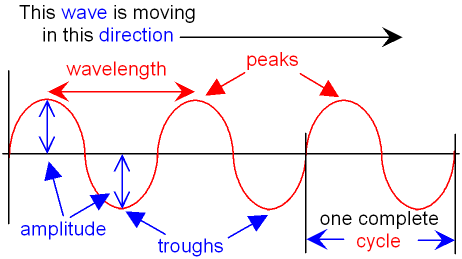# Amplitude in the Musical Context

Amplitude in the Musical Context
At the very basic level, sounds of music are made up of primarily two different properties. Both properties are directly related to the vibration of soundwaves. These properties are Frequency and Amplitude. This article will focus on Amplitude.

To understand Amplitude, one must first understand what a soundwave is. The soundwave is made up of vibrations that go through cycles over time. As we increase the amount of cycles that occur in a period of time, we cause the frequency to rise. Though the frequency is changed, this does not effect how ‘loud’ the sound will be. This is because amplitude is based on how much change of the vibration occurs in an individual cycle.

Here is a diagram of a single cycle in a soundwave:A single cycle of a soundwave can be analyzed horizontally and vertically. The horizontal measurement of a cycle is defined as the wavelength (represented by λ). The vertical measurement, from the top of a peak to the center line, is the amplitude (represented by A). Amplitude is what determines how loud a soundwave is.
As the amplitude of a soundwave is increased the volume created by the soundwave also increases.

For example, the soundwave on the right will be louder than the left because of the degree of change in an individual cycle.Understanding amplitude at a physics level helps to understand why music sounds the way it does.